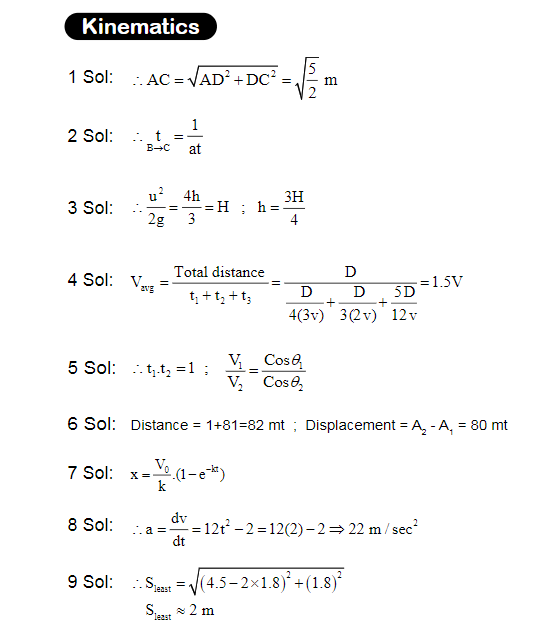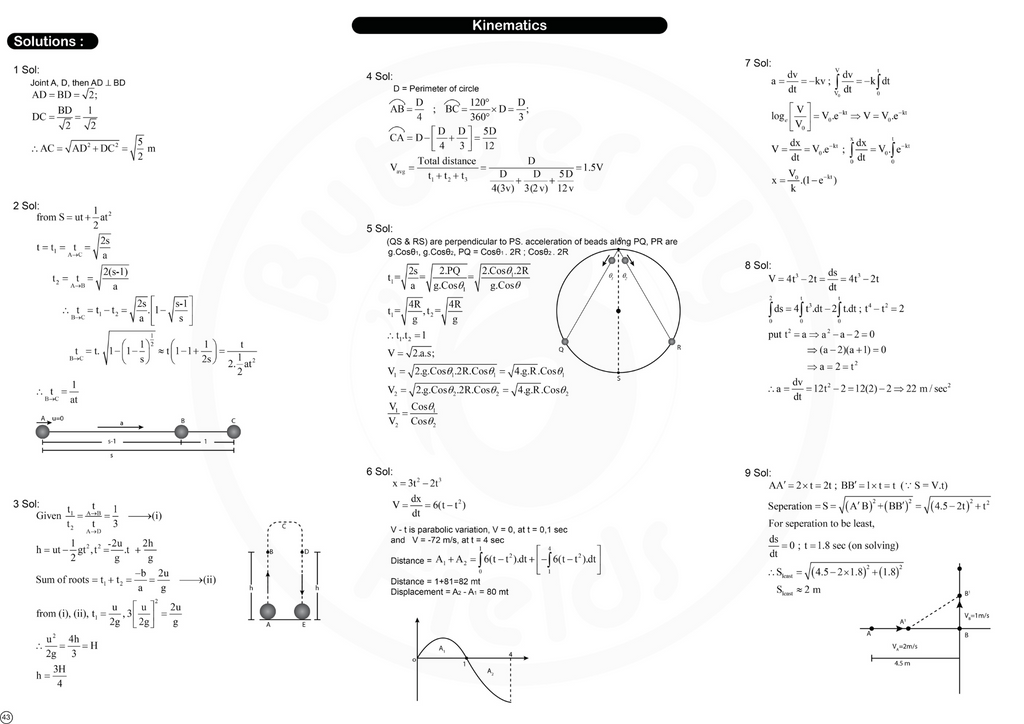## Concept map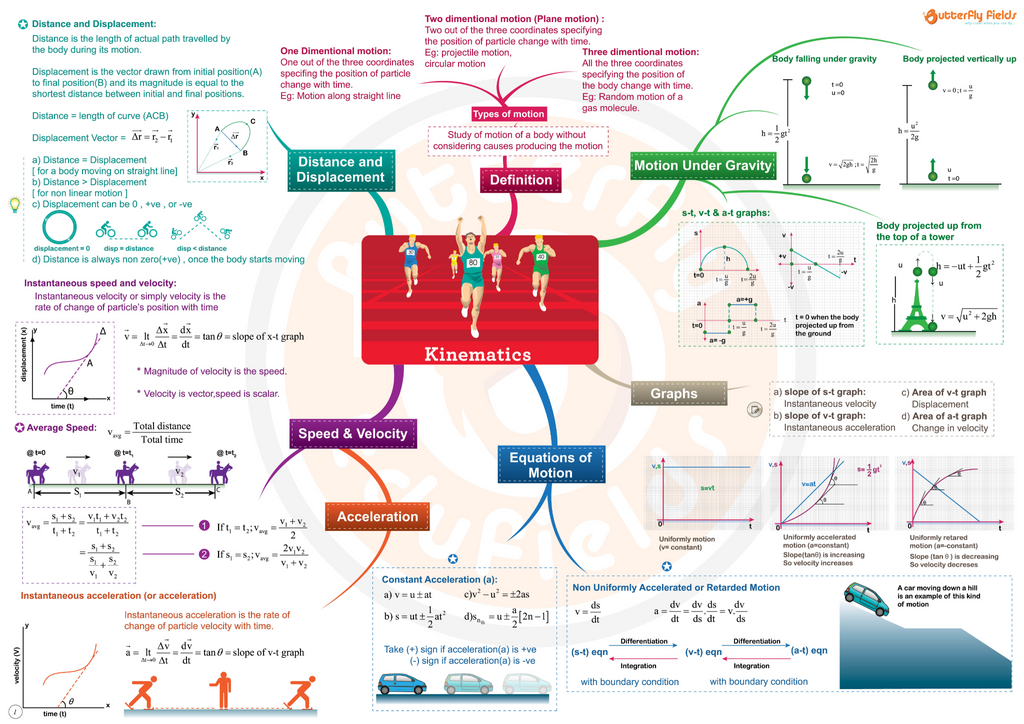# Questions

1) In the figure shown, ADB is a semi circular track of radius = 1m. When a body travels along A → O→ B → C, it suffers ----------disp- -lacement (’C’ is the midpoint of BD)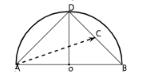2) A body starts from rest and moving along a straight line covers a very very large distance with uniform acceleration (a) in time (t). The time taken to cover last metre of its journey is -------

3) A particle is projected vertically upwards and it attains a maximum height (H). If the ratio of times to attain a height h is 1/3, then h = --

4) Figure shows a particle starting from point A, travelling up to B with speed 3 V, then up to C with 2 V and finally back to A with 3 V. It’s average speed is = ----------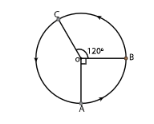5) Two beads start sliding along two wires (PQ, PR) arranged as ch- -ords of a vertical circular ring of radius ‘R’. They reach other ends of the wires in times t1, t2 with velocities v1, v2. then t1 / t2 = ---------- V1 / V2 = ----------

6) The position of a particle moving along x-axis depends on time (t) on, x = (3t2 -2t3) mt. Plot v - t graph and find distance, displacement of the particle during, t = 0 to 4 sec.

7) A particle starts with velocity V0. It’s acceleration (a) and velocity (v) are related as a = - kv [k → constant]. Express displacement as a function of time (t)

8) A body starts from origin and travels along x-axis such that the vel- -ocity (V) at any time (t) is, V = (4t3 -2t) m/sec. What is its accelera- -tion when it is 2 mt away from the origin ?

9) Two bodies are at points A,B on straight line are 4.5m apart. The body from A is moving towards B at a constant speed 2 m/sec, and the body from B moves perpendicular to AB at 1 m/sec. Find the least seperation between them.